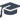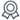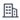• ` 中文

博士生导师
• 招生学科专业：
力学 -- 【招收博士、硕士研究生】 -- 航空学院
机械 -- 【招收博士、硕士研究生】 -- 航空学院
•学位：工学博士学位
•职称：教授
•所在单位：航空学院

Probability distributions control for multi-input multi-output stationary non-Gaussian random vibration test

JOURNAL OF VIBRATION AND CONTROL

Inverse system multi-input multi-output non-Gaussian probability distribution vibration control

An inverse system method for multi-input multi-output stationary non-Gaussian random vibration test is proposed in the paper to control the response characteristics both in time-domain and frequency-domain simultaneously. Hence, the control objectives are not only the traditional response power spectral densities but also probability distributions. The control of the probability distributions is more comprehensive and reasonable than the control of kurtoses in order to duplicate the time-domain characteristics of the measured data because kurtoses provide only partial behaviors of the probability distributions. The nonlinear transformation process is introduced to generate non-Gaussian random signals with specified probability distributions. To obtain the desired vibration environments for the test, the reference response signals are synthesized first by the reference spectra and probability distributions, and then the coupled drive signals are generated via the inverse system in the time domain. The close loop correction algorithms are implemented to update the drive signals, actually to update the reference response signals according to the deviations between the references and the measured responses. Finally, a numerical example by a cantilever beam and a biaxial vibration test are carried out and the results demonstrate the effectiveness and feasibility of the proposed method.
ISSN号：
1077-5463

2018-11-01

,,陈怀海,陈怀海,陈怀海,陈怀海,陈怀海,陈怀海,陈怀海,陈怀海,陈怀海,陈怀海

Zheng, Ronghui,贺旭东,郑玮

2018-11-01